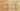EqualsComparesReferences

Misconception:

The equals(Object) method checks whether two object references refer to the same object.Incorrect

o.equals(p) compares the references stored in the variables o and p

Correct

o.equals(p) compares the objects referred to by variables o and p

CorrectionHere is what's right.

The equals(Object) method does not necessarily compare references; in fact, the intent of this method is to compare object’s values. To check whether two object references refer to the same object, use the == operator instead:

Pacman a = new Pacman(1, 2);
Pacman b = new Pacman(1, 2);
assert !(a==b); // a and b are NOT the same

The equals(Object) method, which is introduced in java.lang.Object and often is overridden in subclasses, usually checks whether the two objects have an equal state (they may be two different objects, but their instance variables will have equal values).

public class Pacman {
private int x;
private int y;
public Pacman(int x, int y) {
this.x = x;
this.y = y;
}
public boolean equals(Object o) {
if (o instanceof Pacman) {
Pacman other = (Pacman)o;
return other.x==this.x && other.y==this.y; // compare fields
} else {
return false;
}
}
}

Pacman a = new Pacman(1, 2);
Pacman b = new Pacman(1, 2);
assert a.equals(b); // a and b are equal

Note that equals(Object) can be overridden in any class, and while it is customary to compare the state (by comparing the fields) of the two objects, one can also just compare the references (i.e., use return this==other to implement the equals method).

The precise specification of the semantics can be found in the Object.equals(Object) API documentation.

ValueHow can you build on this misconception?

This misconception is a good opportunity to discuss the difference between the general concepts of structural equality (equal objects, i.e., two objects with equivalent state) and referential equality (same object).

Some languages, e.g., Kotlin, provide separate operators for the two kinds of equality. Java only provides an operator for reference equality (==) and expects developers to call the equals(Object) method to test for structural equality.